# ICSE Class 10 Chemistry Question Paper Solution 2018

Exam preparation is incomplete till the student does not evaluate its performance. The best way to check the exam preparation is by solving the ICSE Class 10 previous year question papers. Here, we have provided the ICSE Class 10 Chemistry Question Paper 2018 along with the Solutions. This ICSE Class 10 Chemistry Question Paper 2018 solution will help students in identifying their weak and strong points. Working on them, students can improve their performance in the board exam. Also, it will directly improve their scores in the exam.

The ICSE Class 10 Chemistry 2018 exam was conducted on 19th March 2018. The exam started at 11 am and students were allotted 2 hours of time duration to finish the paper. Students can download the ICSE Class 10 Chemistry Question Paper Solution 2018 PDF from the link below.

Students can have a look at the ICSE Class 10 Chemistry Question Paper Solution 2018 below: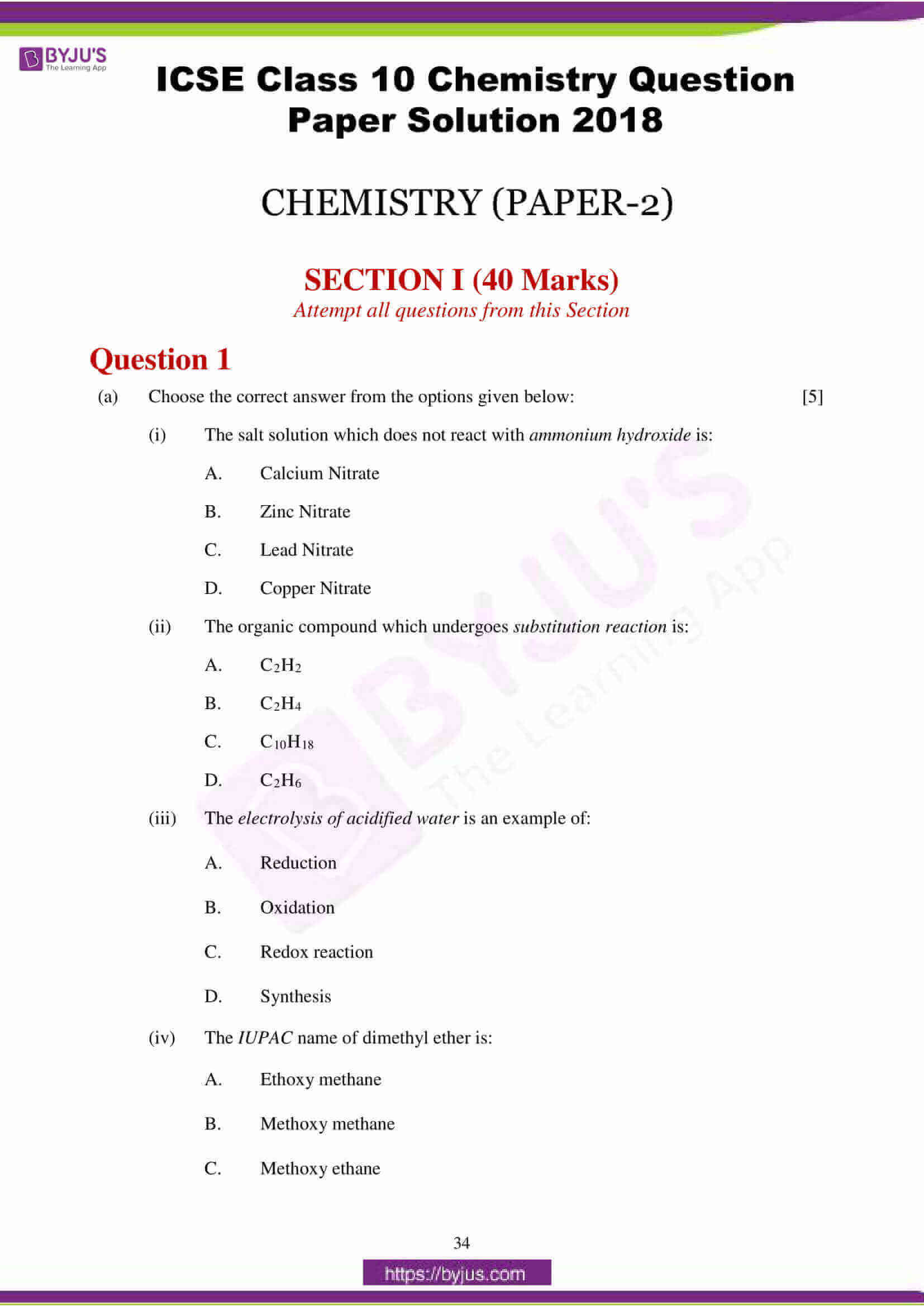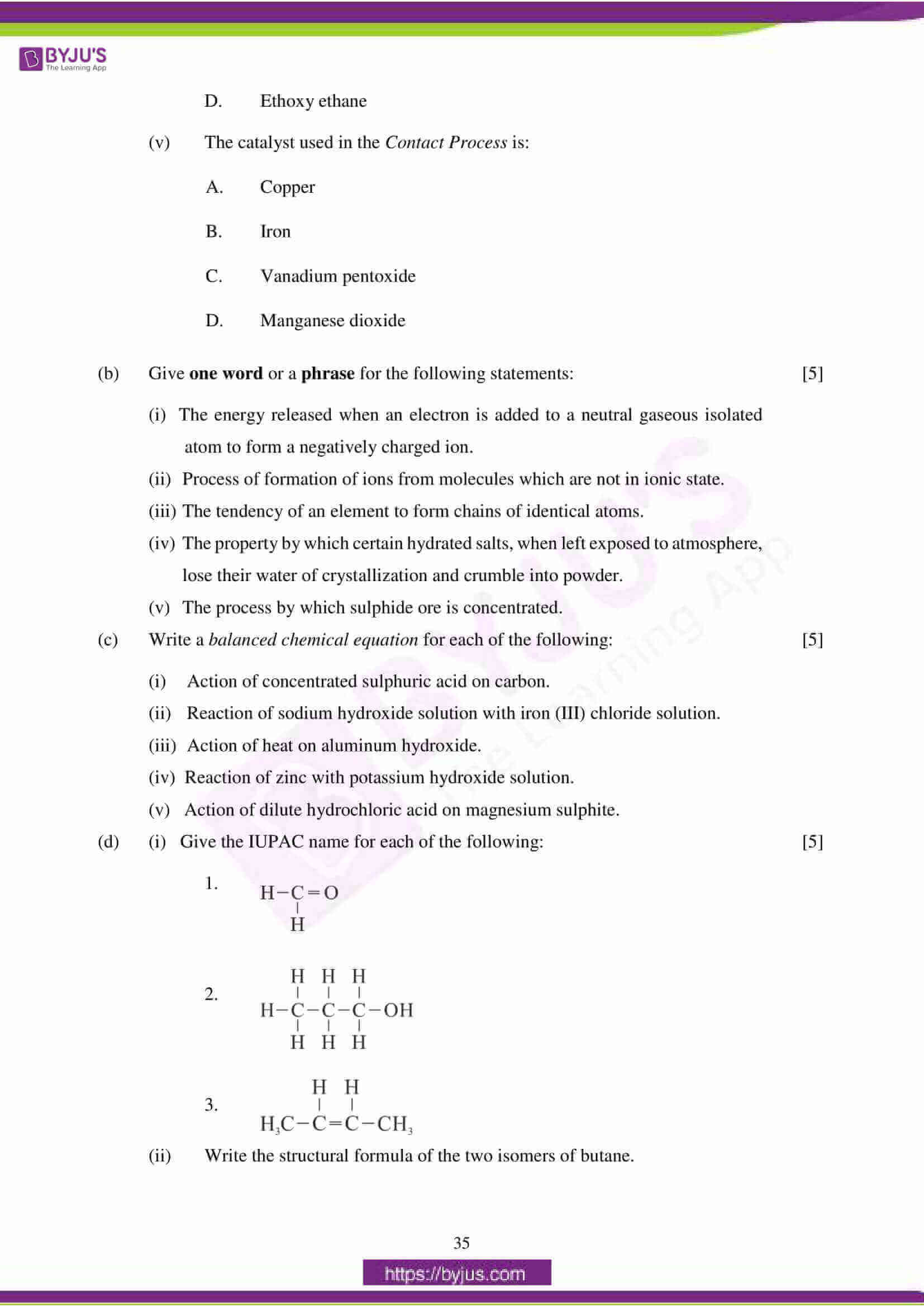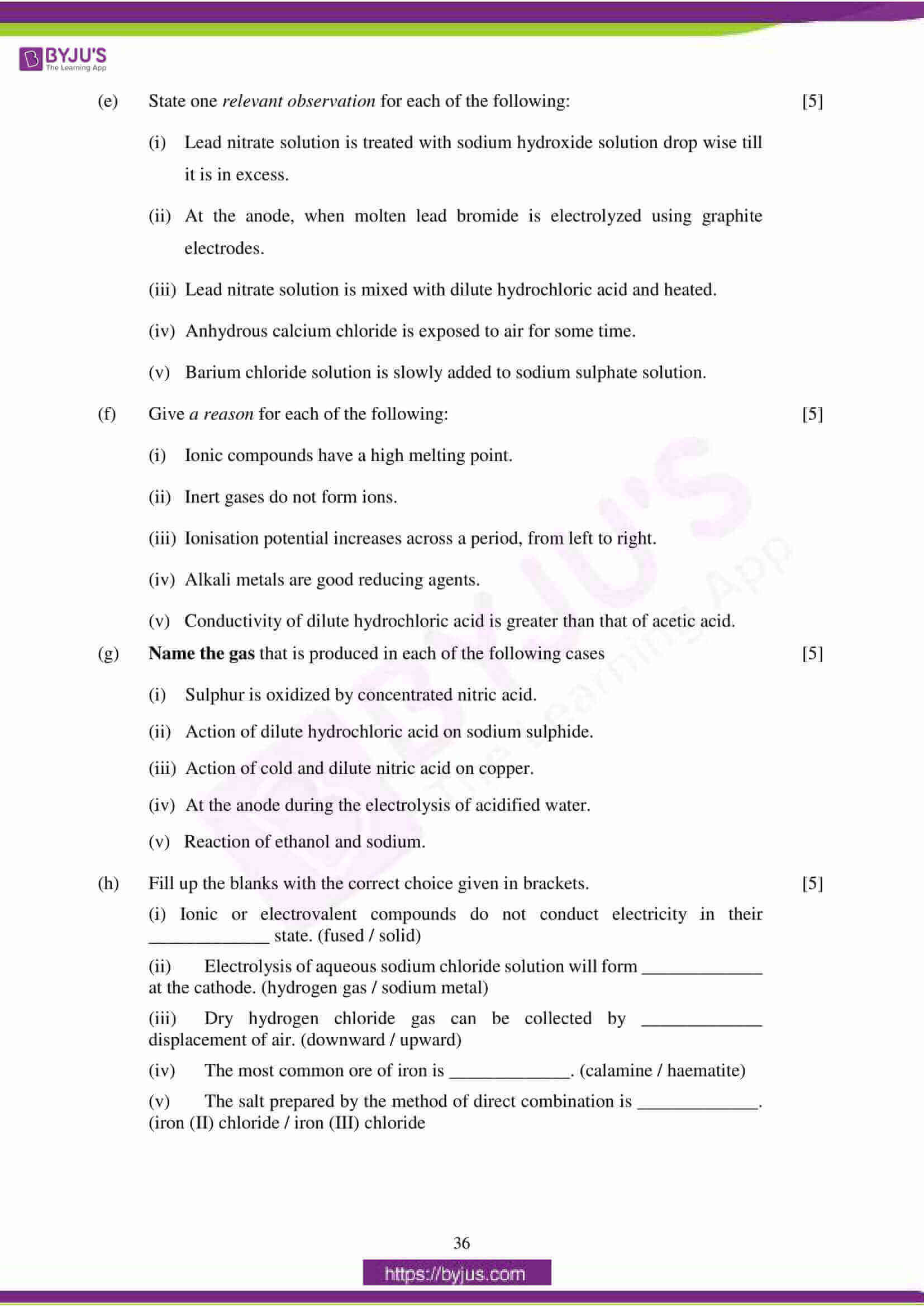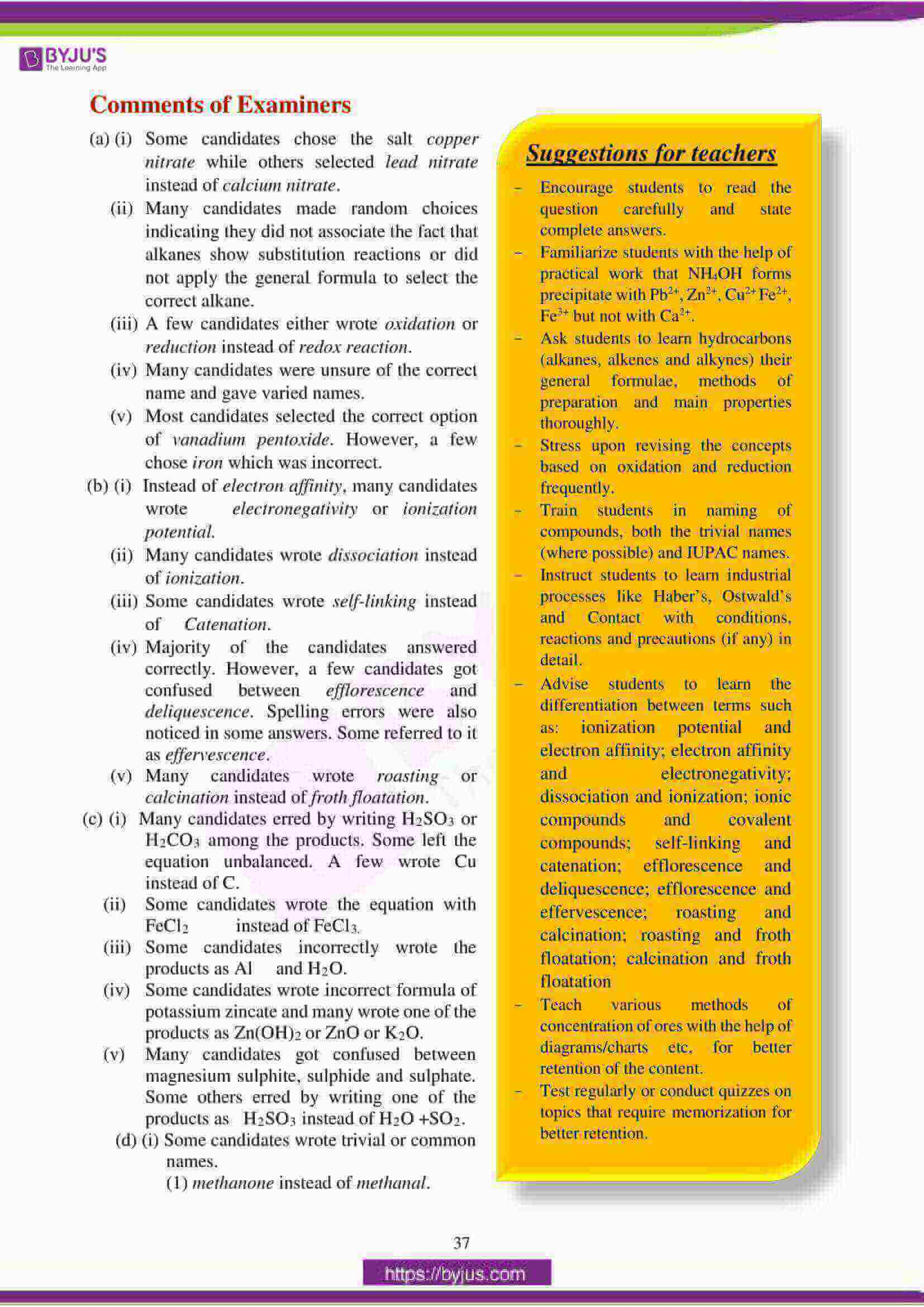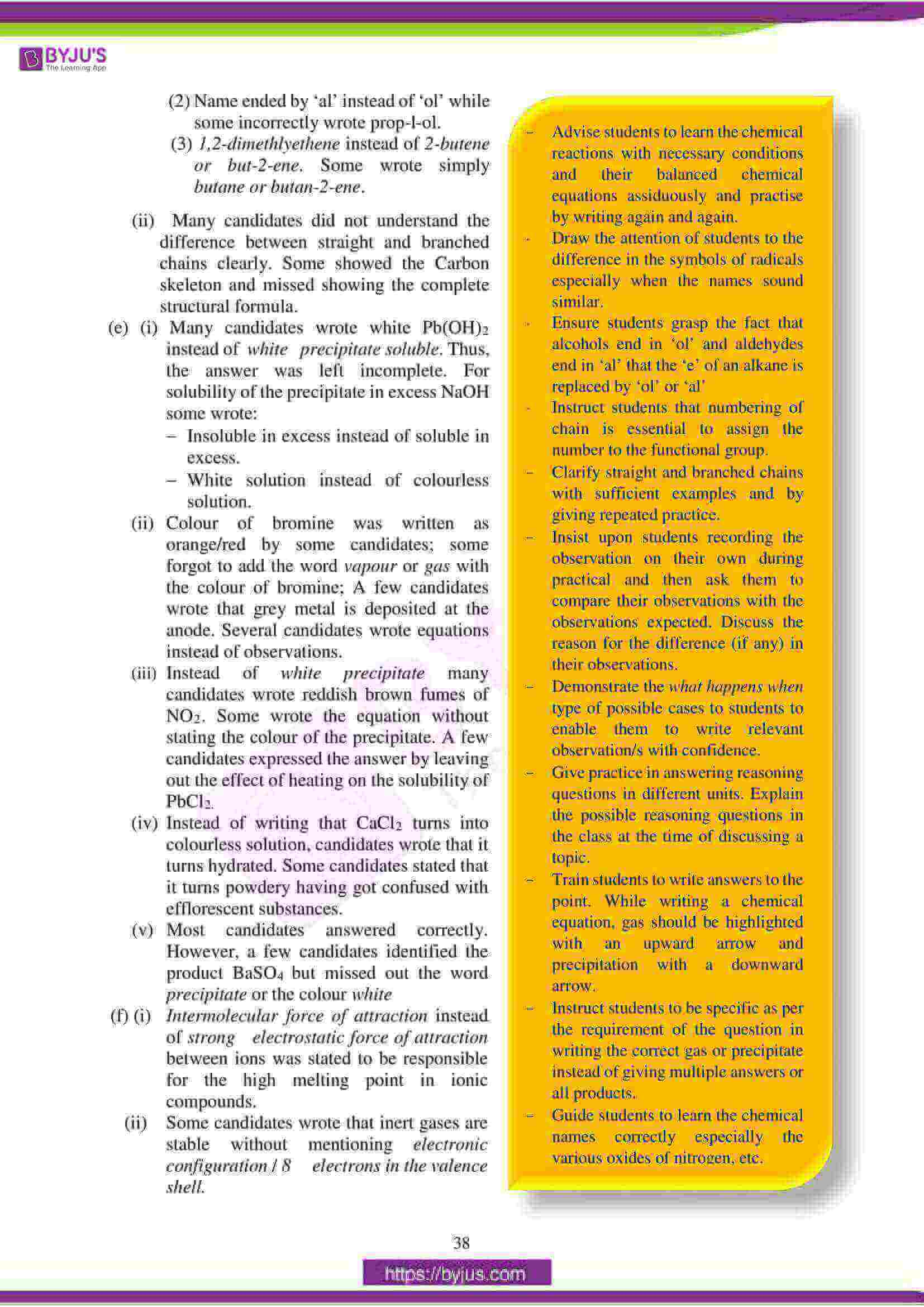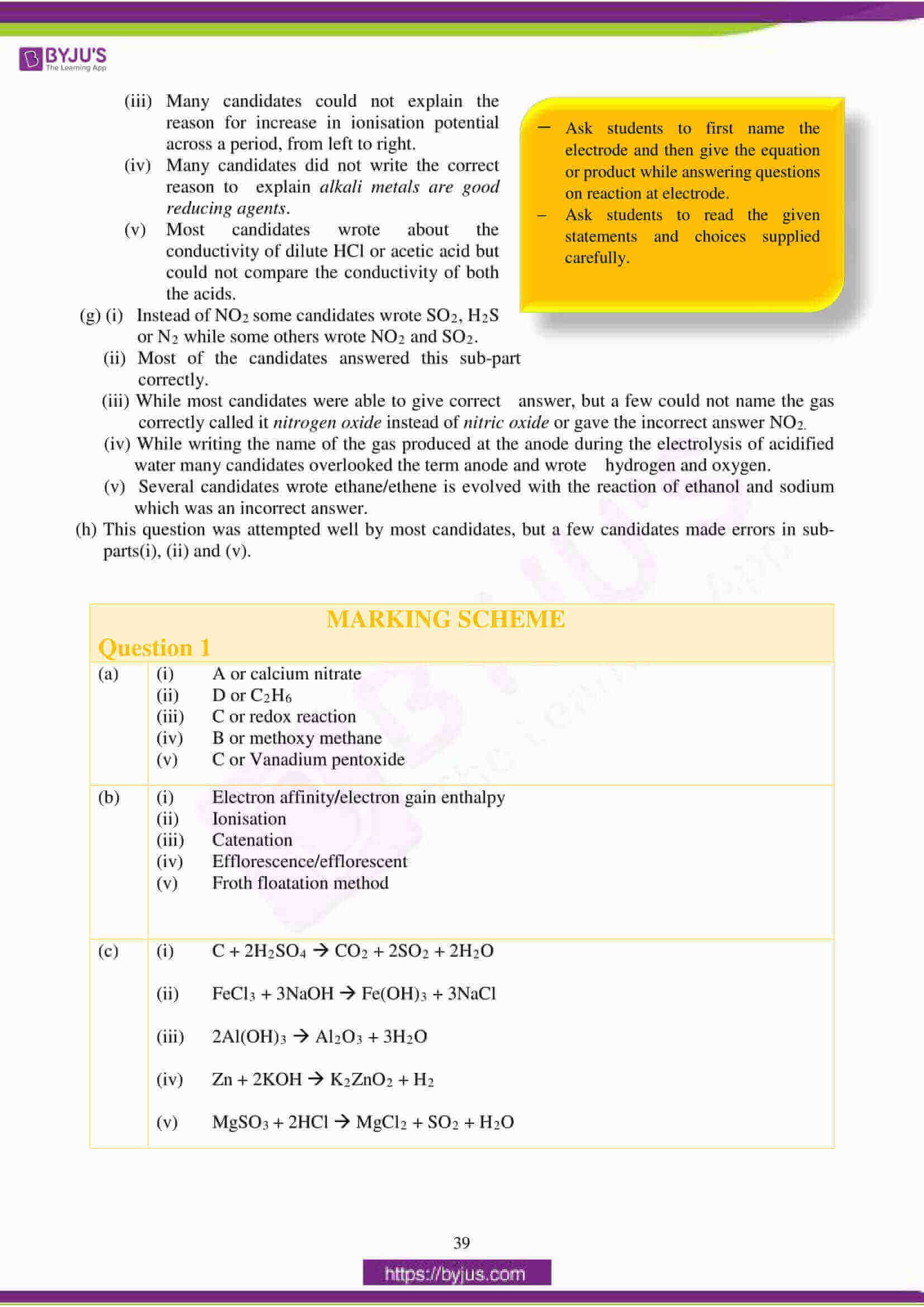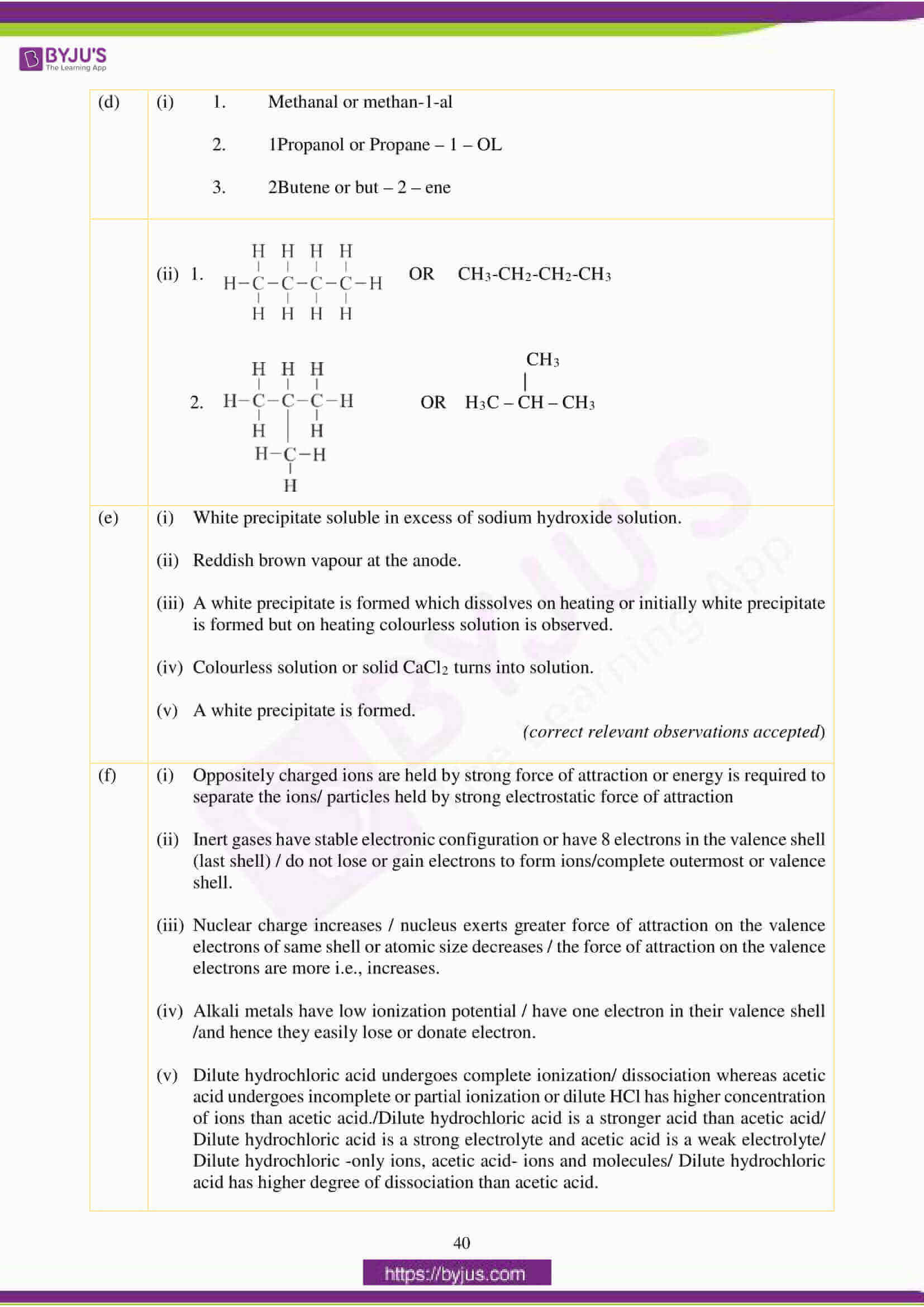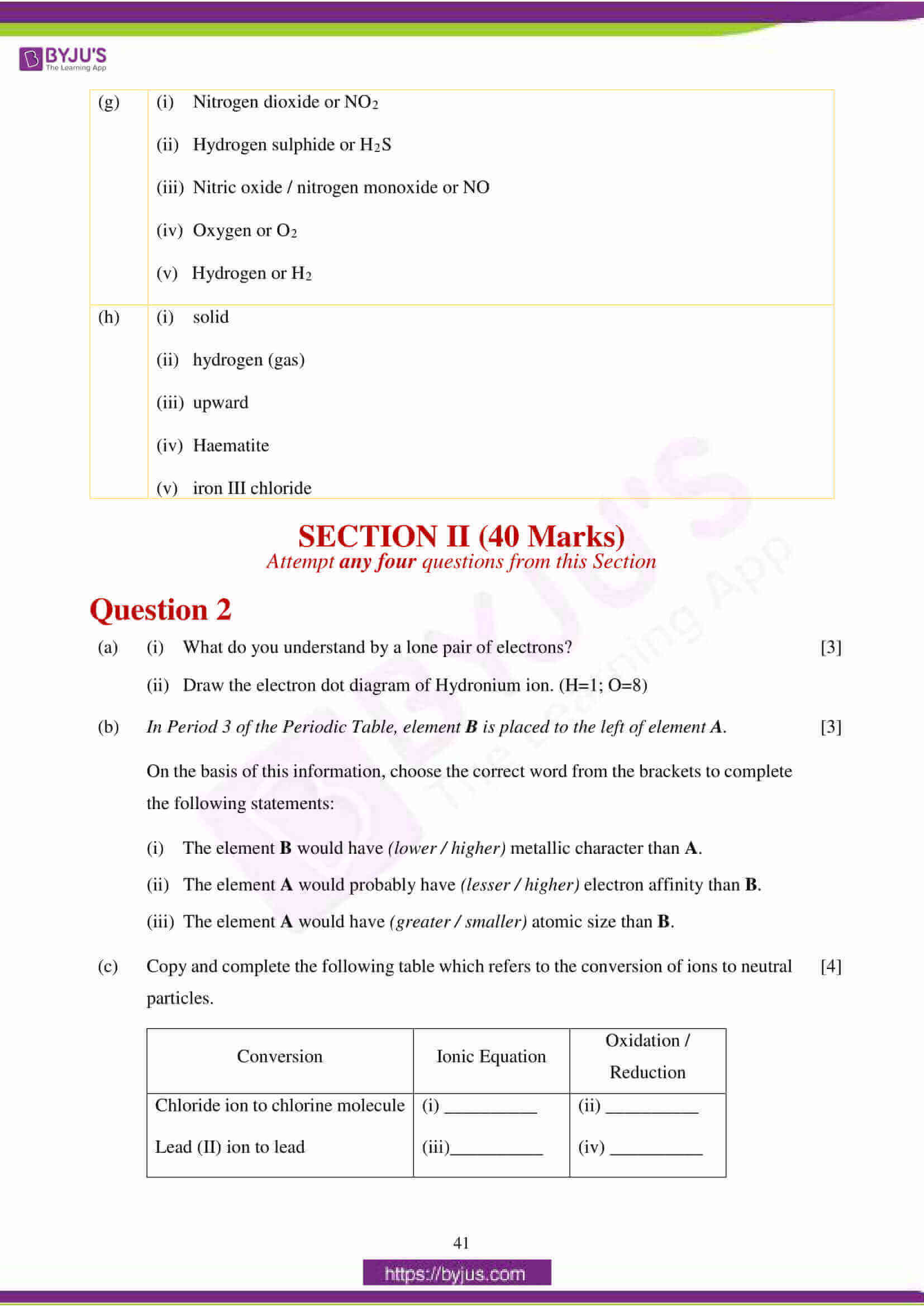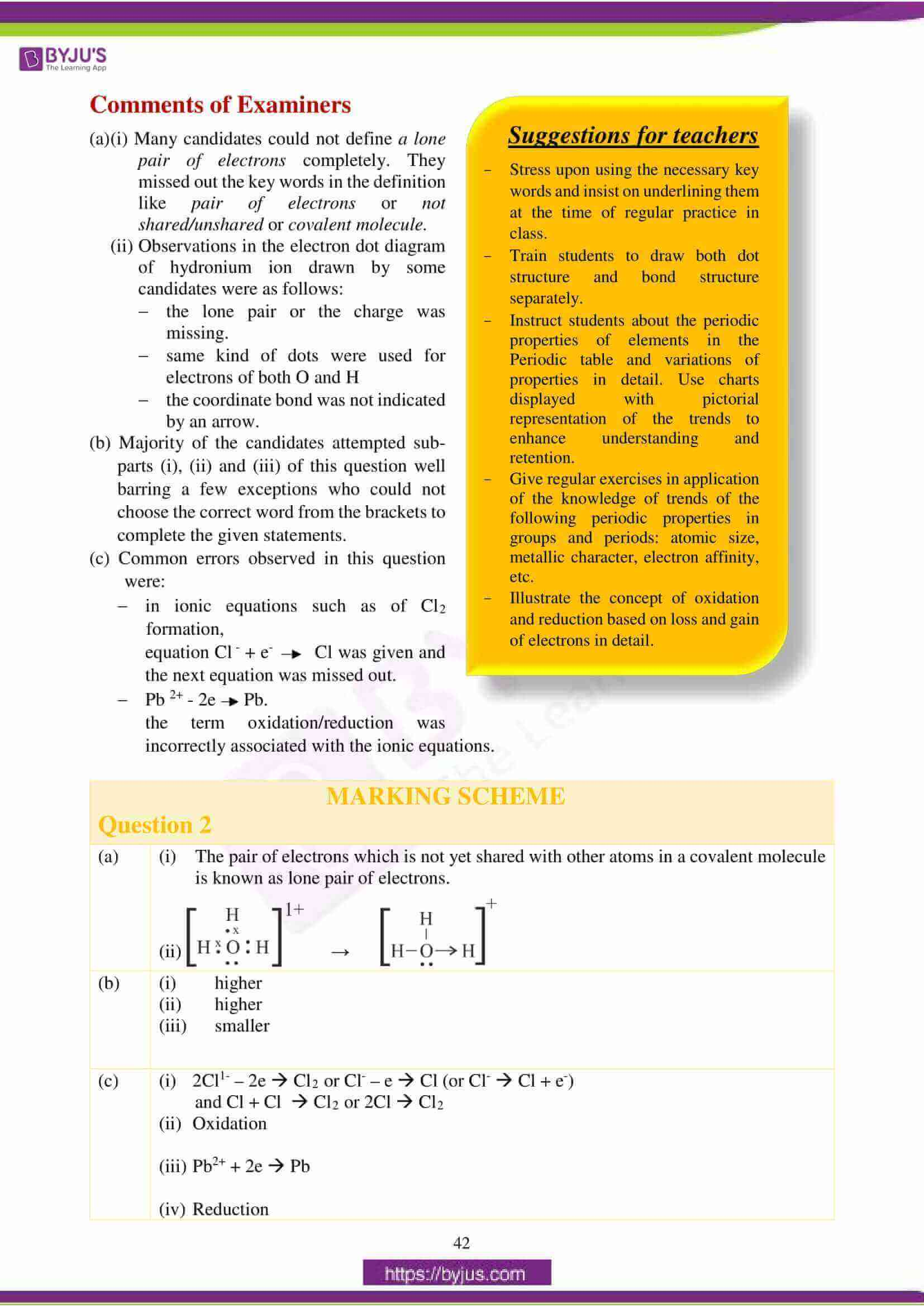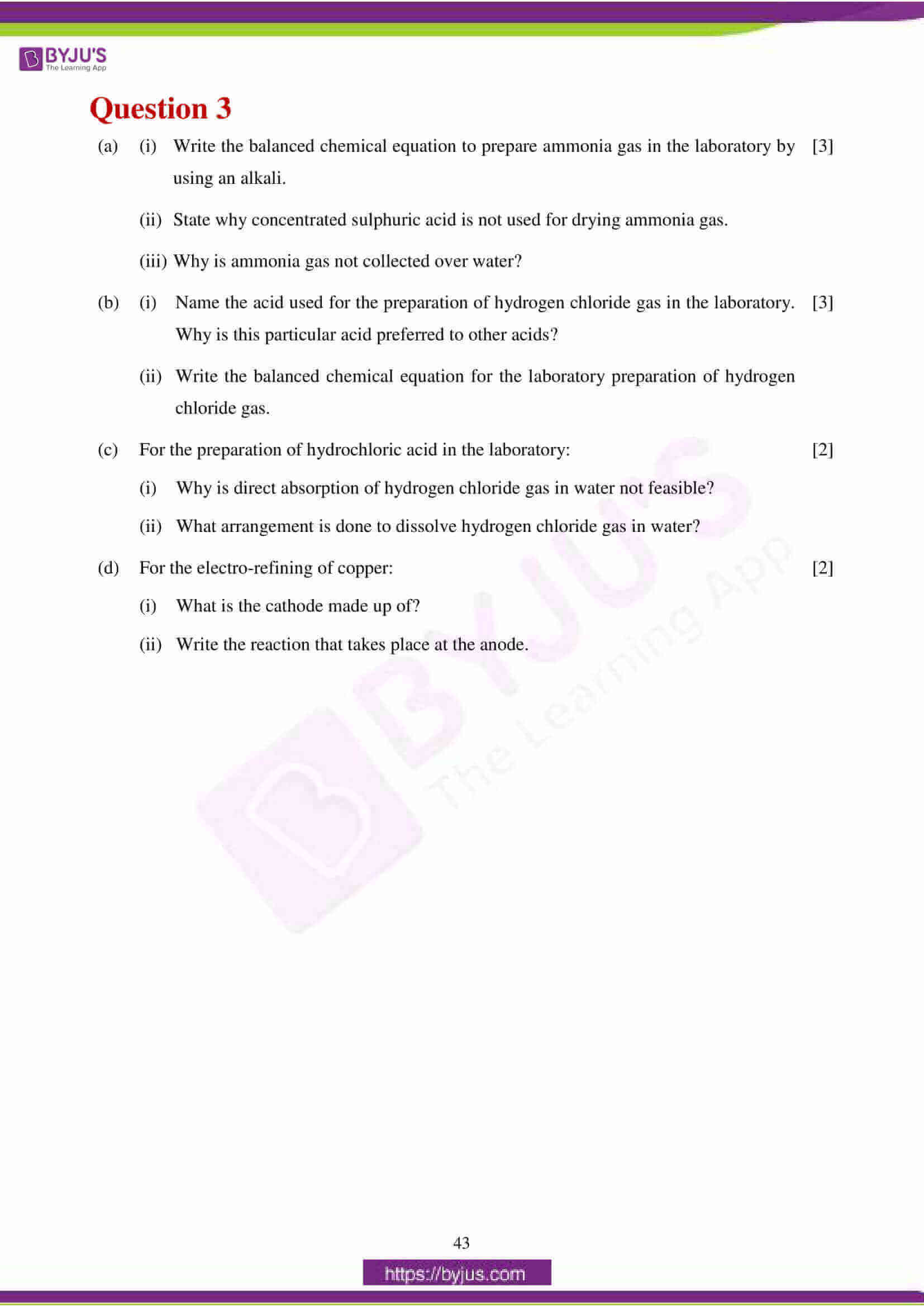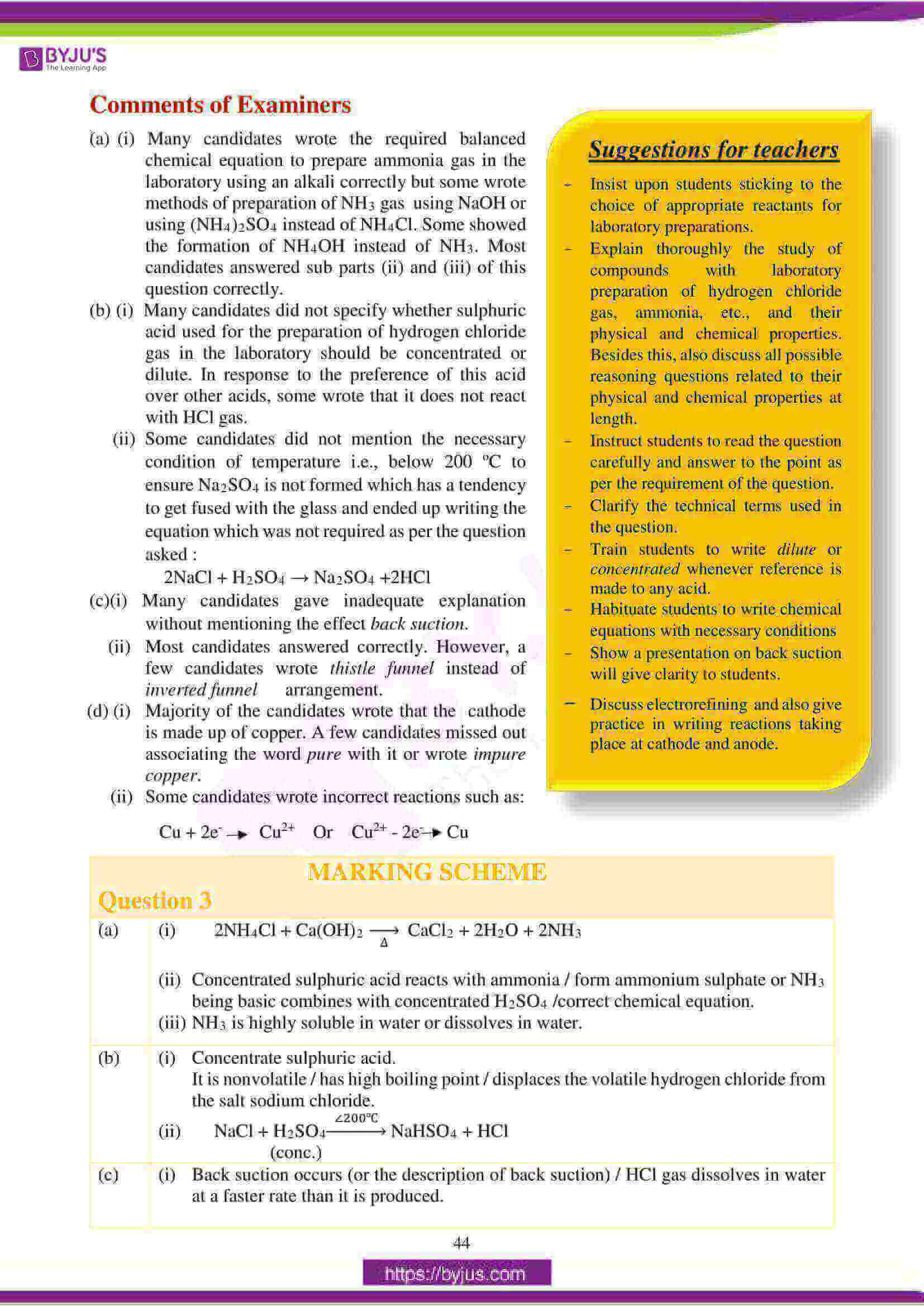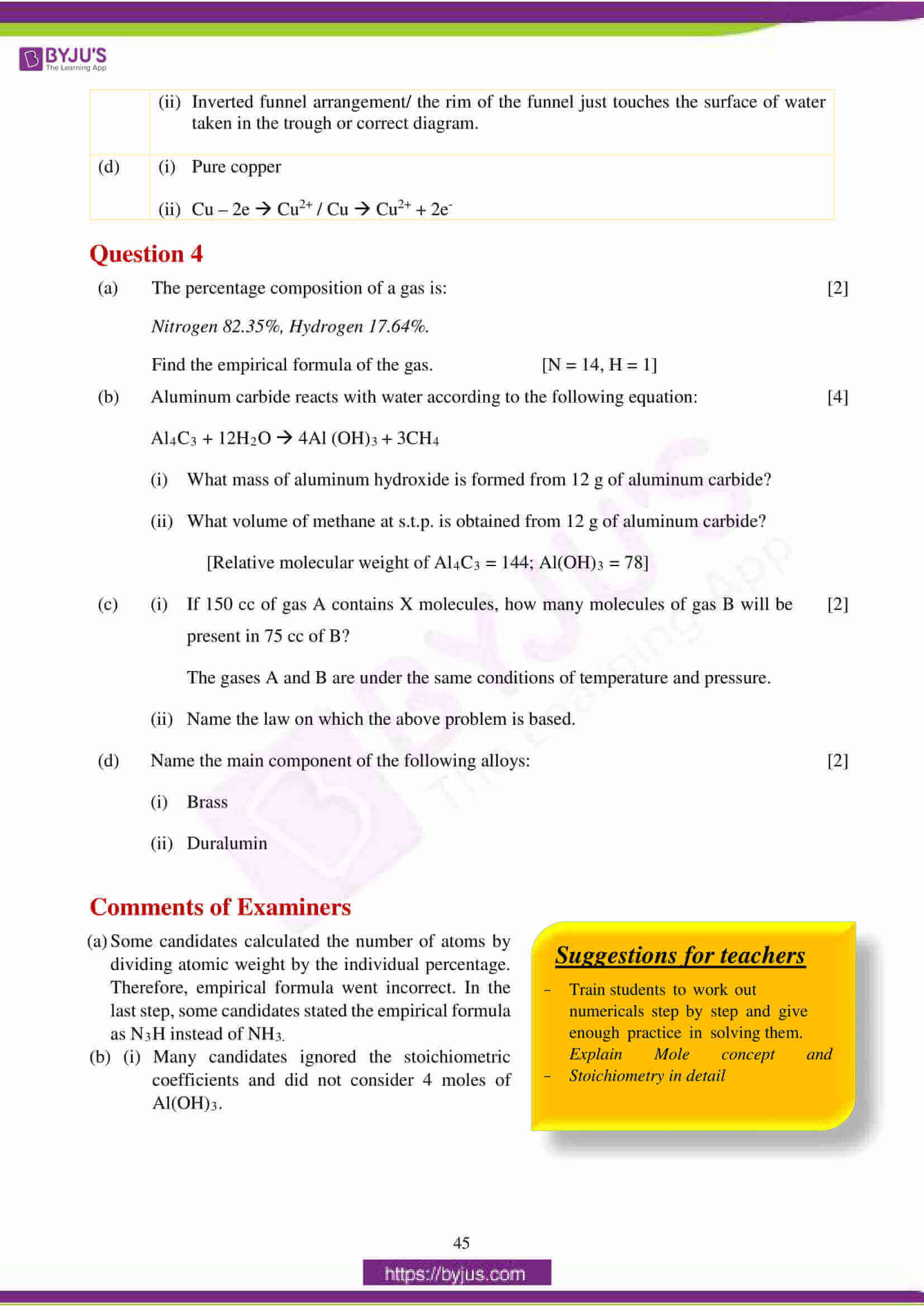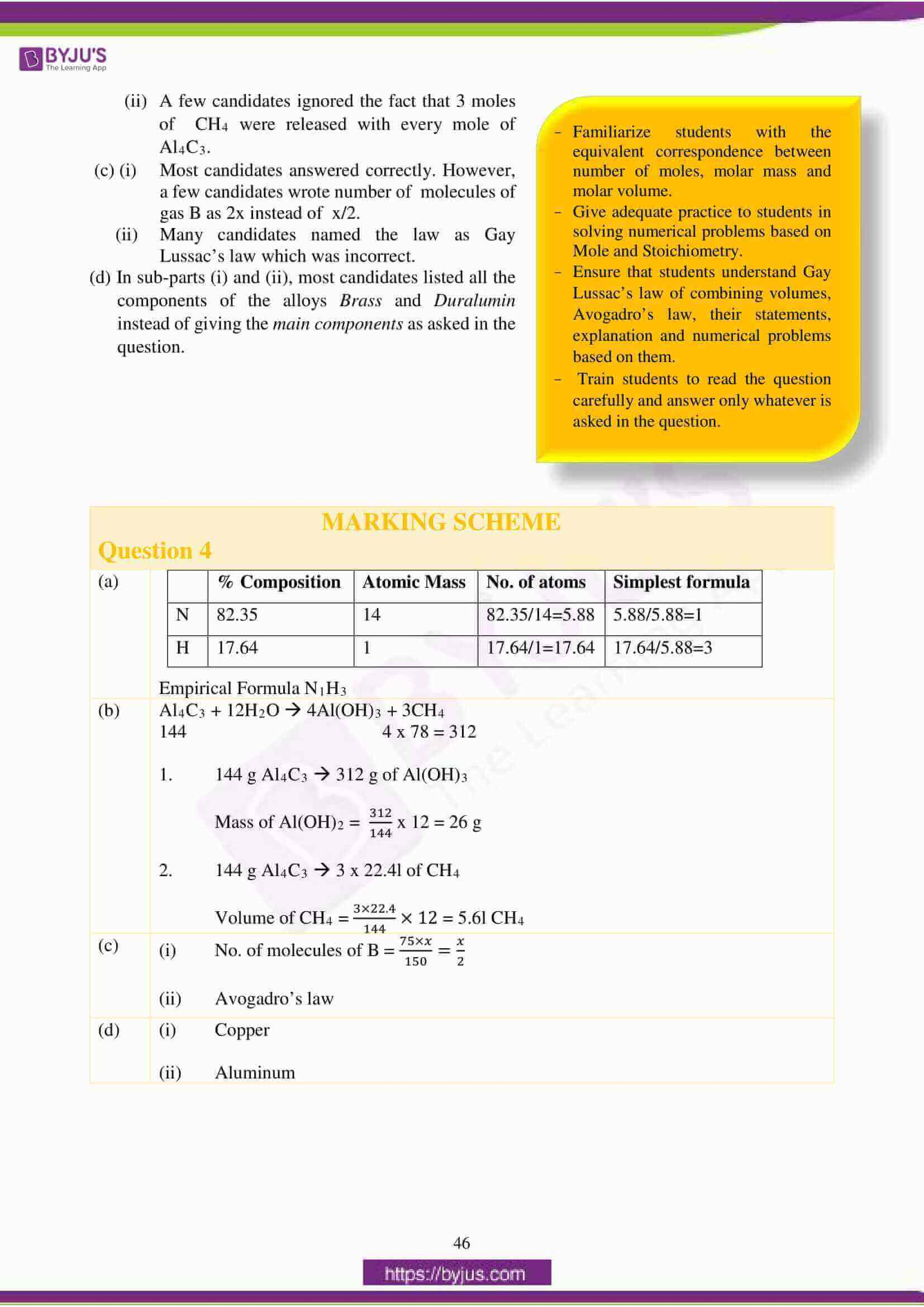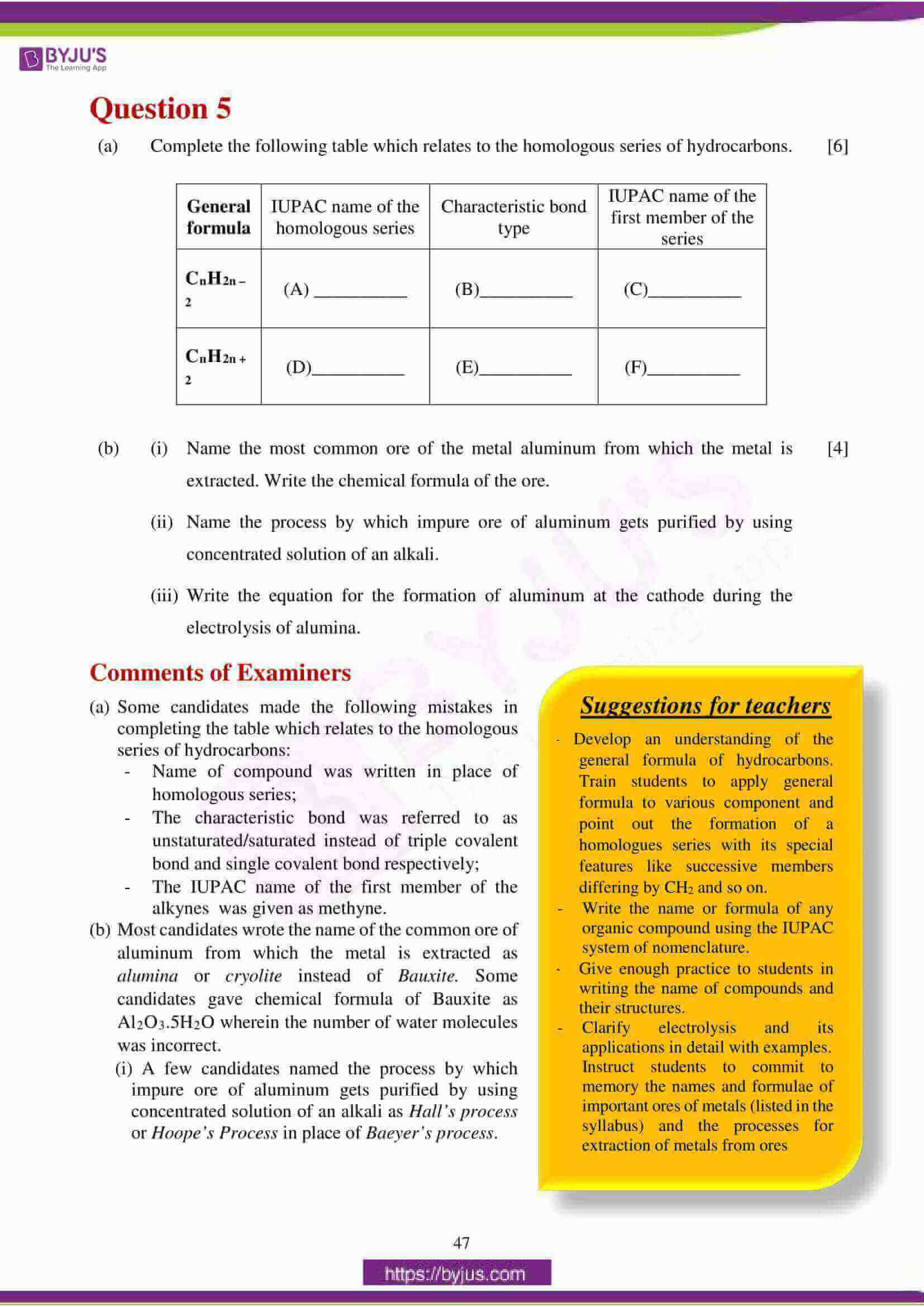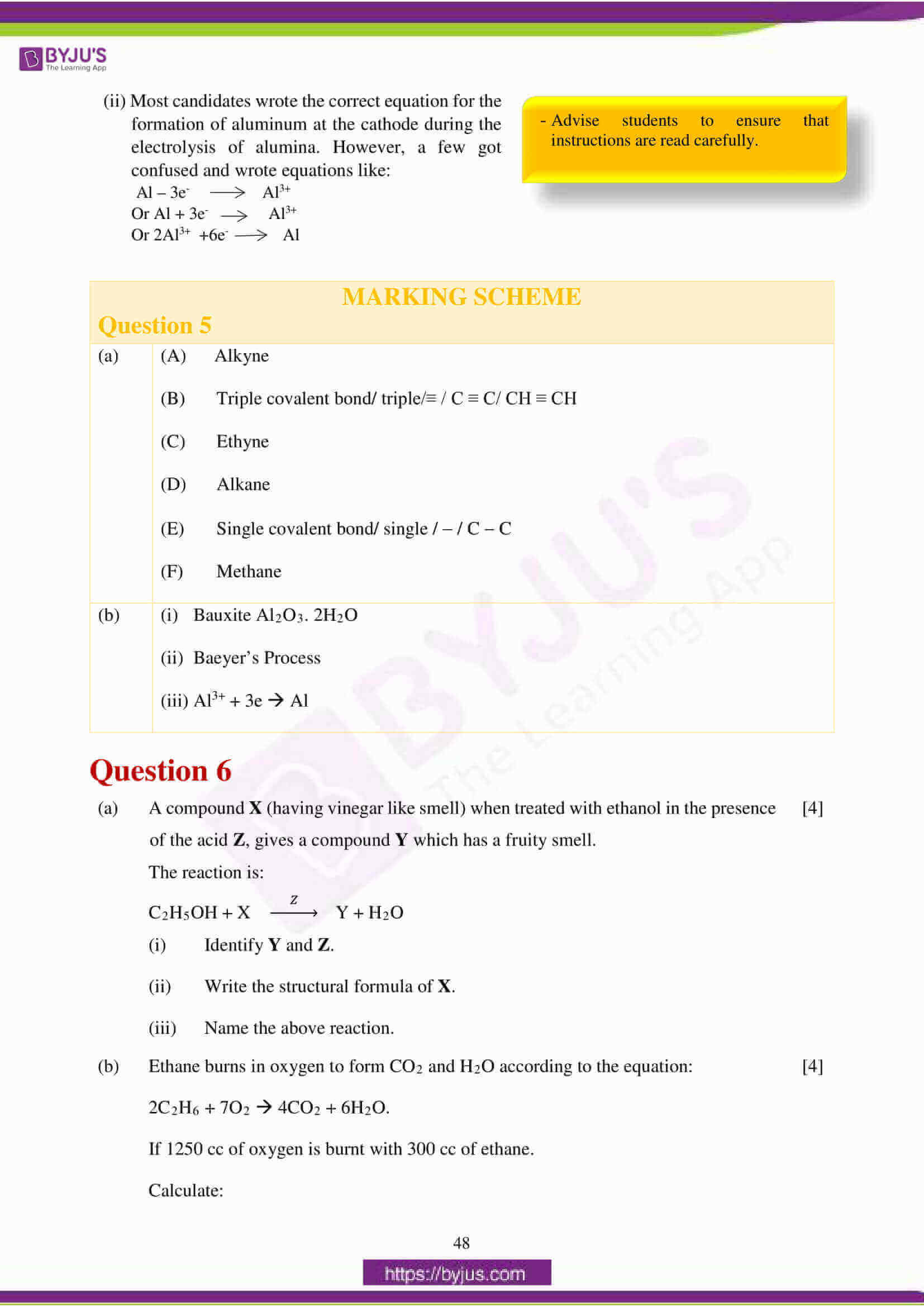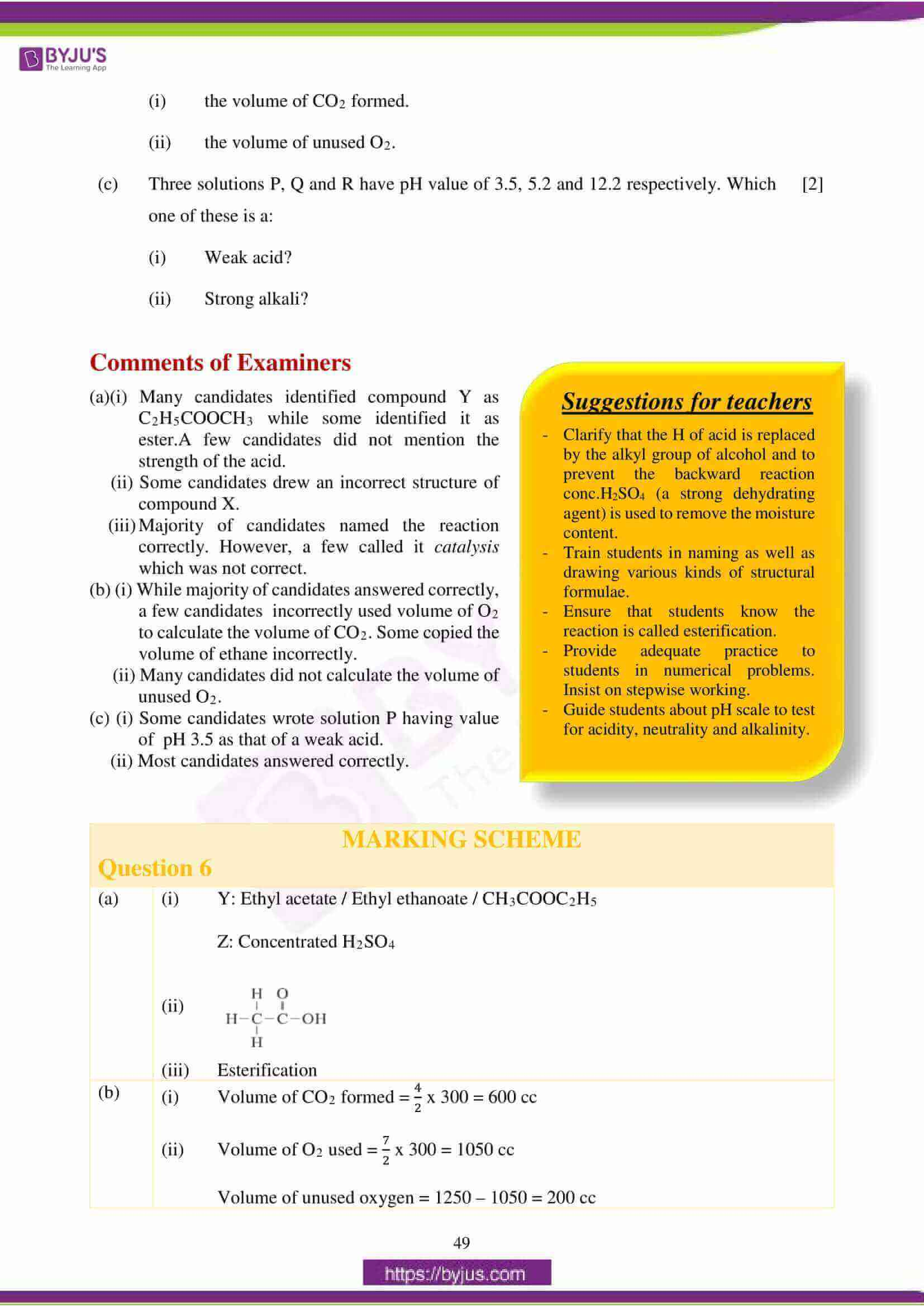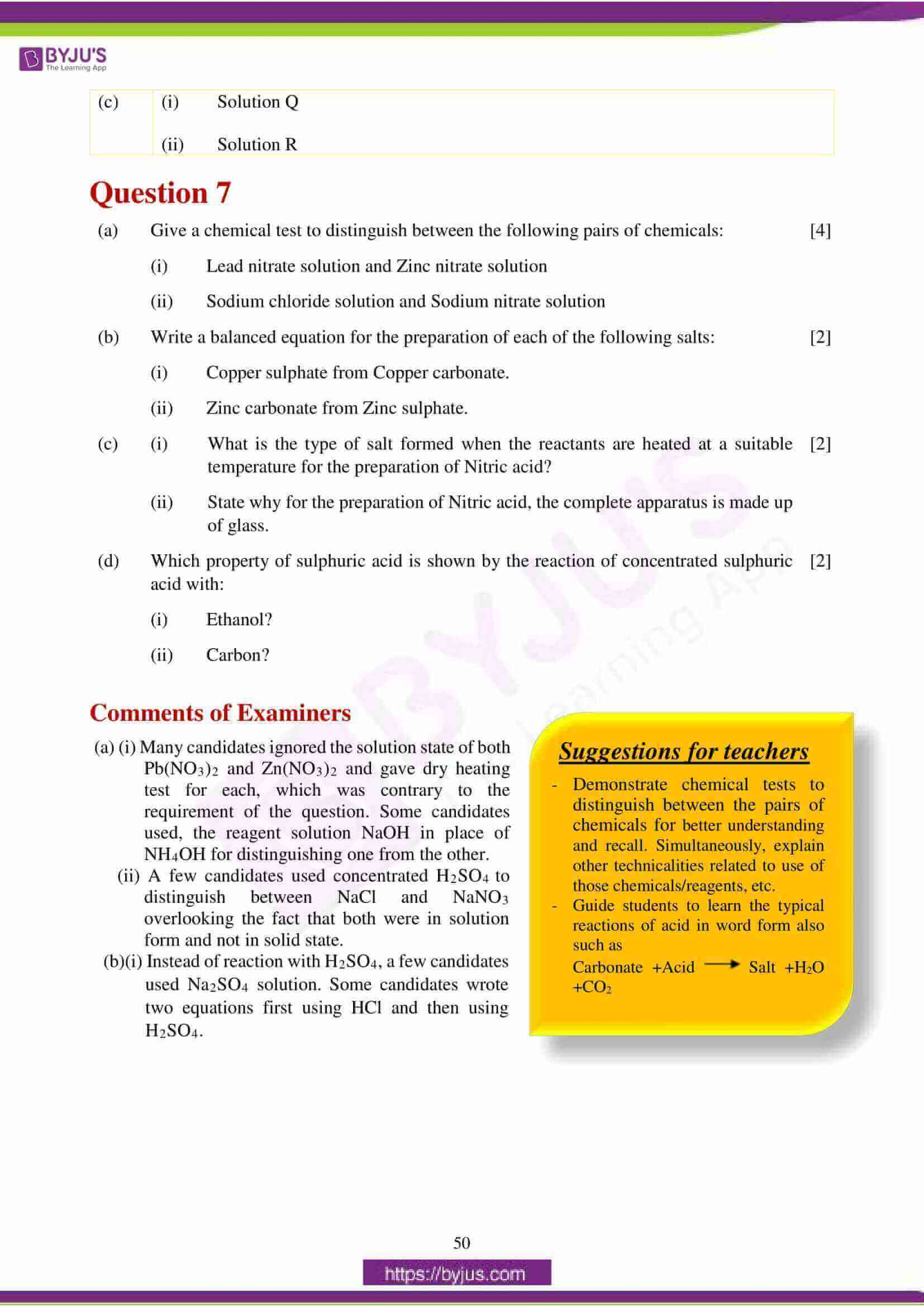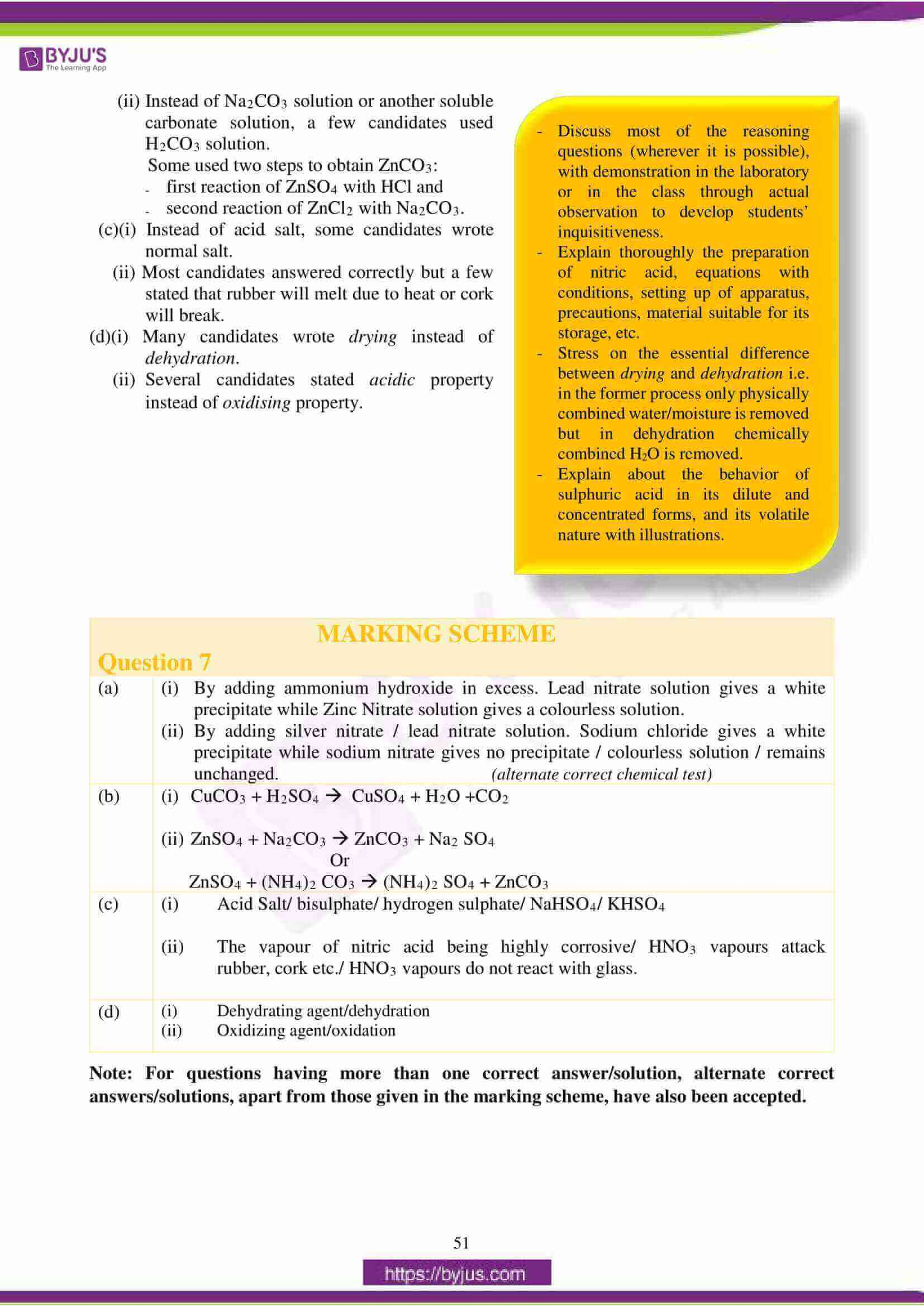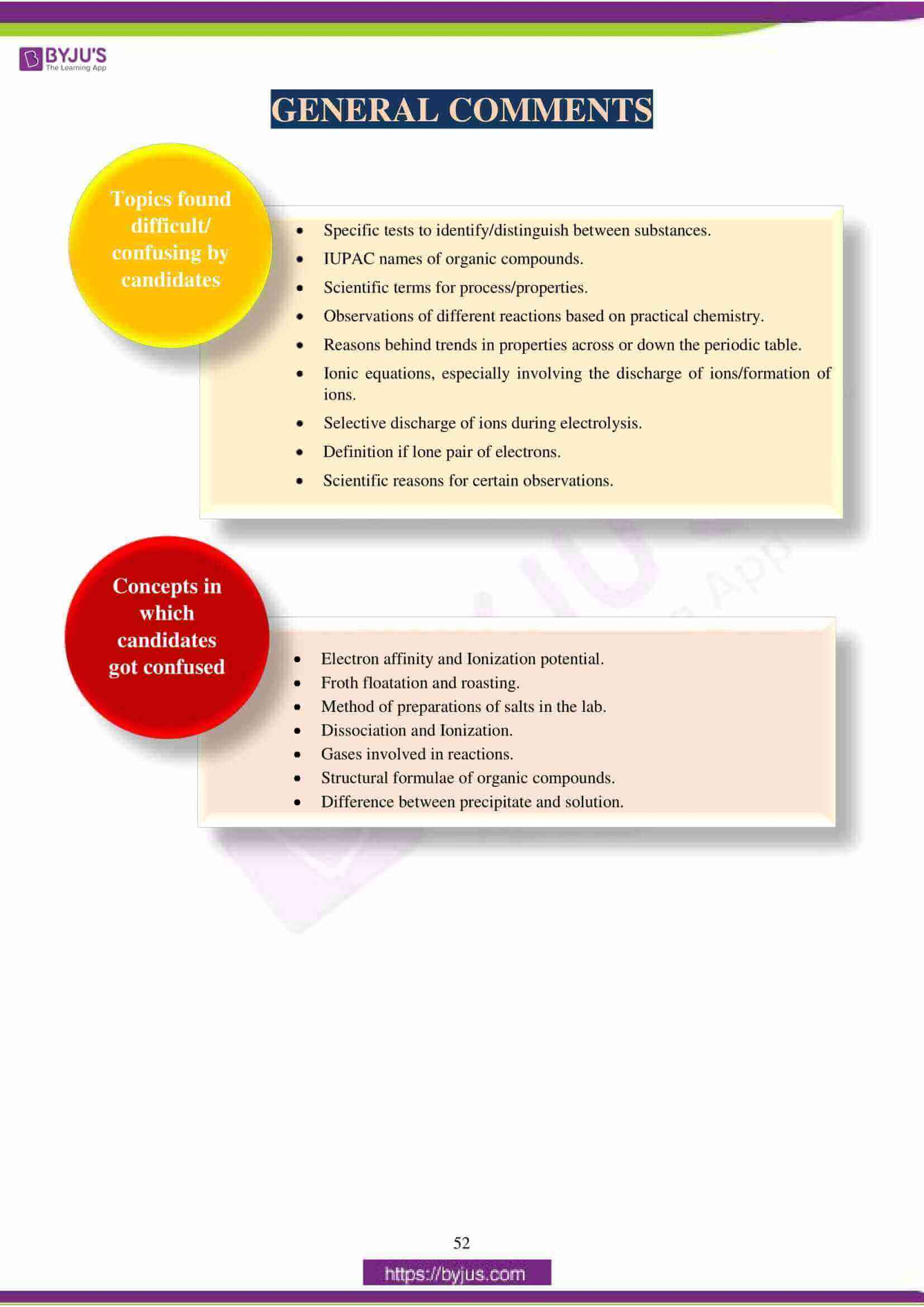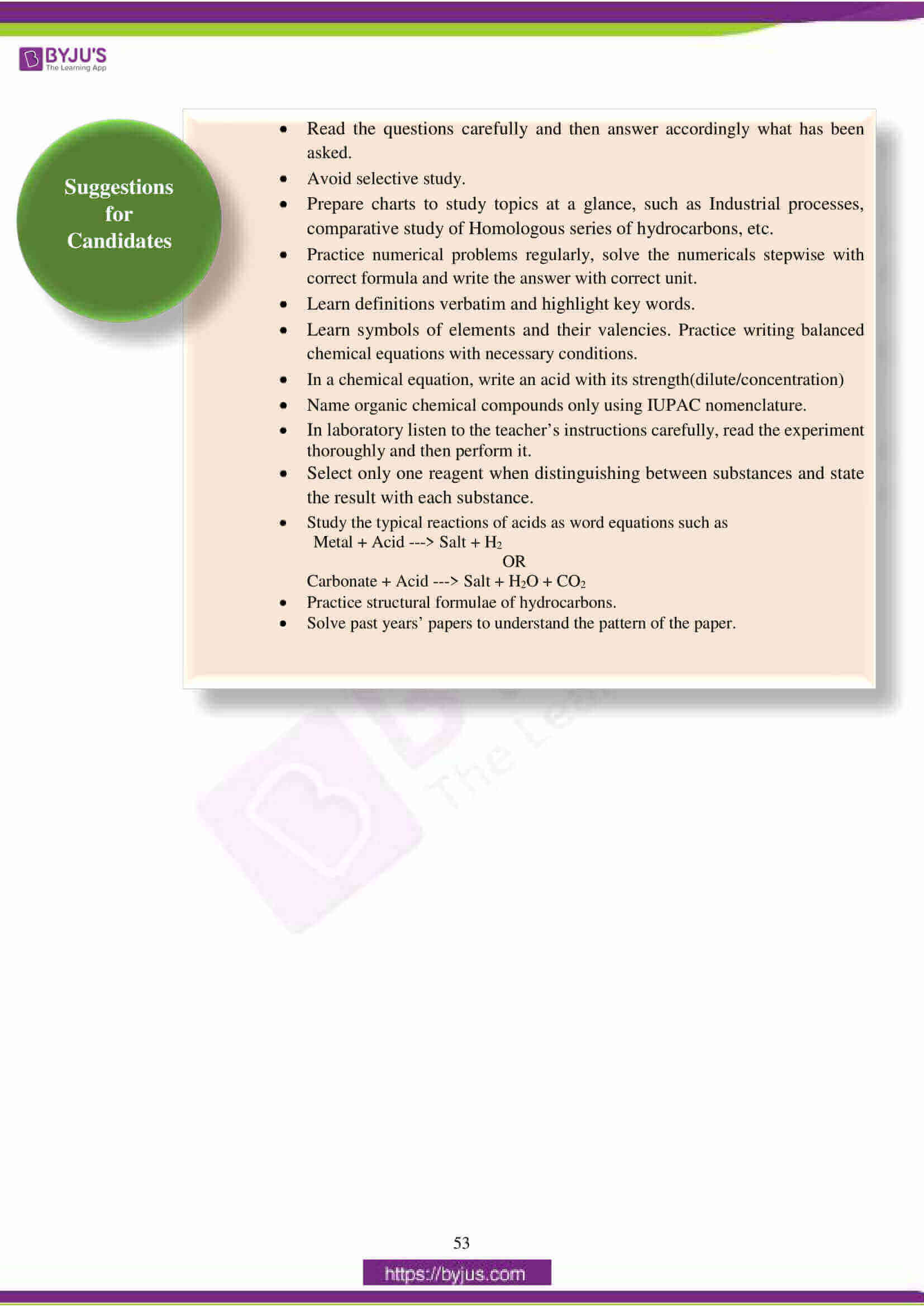## ICSE Class 10 Chemistry (Paper 2) Question Paper 2018 With Solution

### Question 1:

(a) Choose the correct answer from the options given below:

(i) The salt solution which does not react with ammonium hydroxide is:

A. Calcium Nitrate

B. Zinc Nitrate

D. Copper Nitrate

(ii) The organic compound which undergoes substitution reaction is:

A. C2H2

B. C2H4

C. C10H18

D. C2H6

(iii) The electrolysis of acidified water is an example of:

A. Reduction

B. Oxidation

C. Redox reaction

D. Synthesis

(iv) The IUPAC name of dimethyl ether is:

A. Ethoxy methane

B. Methoxy methane

C. Methoxy ethane

D. Ethoxy ethane

(v) The catalyst used in the Contact Process is:

A. Copper

B. Iron

D. Manganese dioxide

(b) Give one word or a phrase for the following statements:

(i) The energy released when an electron is added to a neutral gaseous isolated atom to form a negatively charged ion.

(ii) Process of formation of ions from molecules which are not in ionic state.

(iii) The tendency of an element to form chains of identical atoms.

(iv) The property by which certain hydrated salts, when left exposed to atmosphere, lose their water of crystallization and crumble into powder.

(v) The process by which sulphide ore is concentrated.

(c) Write a balanced chemical equation for each of the following:

(i) Action of concentrated sulphuric acid on carbon.

(ii) Reaction of sodium hydroxide solution with iron (III) chloride solution.

(iii) Action of heat on aluminum hydroxide.

(iv) Reaction of zinc with potassium hydroxide solution.

(v) Action of dilute hydrochloric acid on magnesium sulphite.

(d) (i) Give the IUPAC name for each of the following: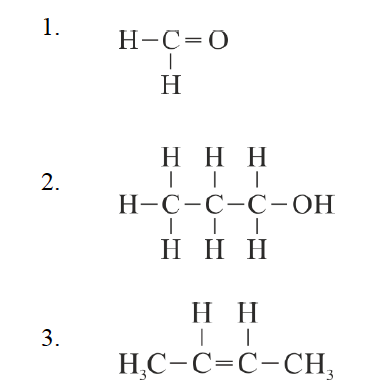(ii) Write the structural formula of the two isomers of butane.

(e) State one relevant observation for each of the following:

(i) Lead nitrate solution is treated with sodium hydroxide solution drop wise till it is in excess.

(ii) At the anode, when molten lead bromide is electrolyzed using graphite electrodes.

(iii) Lead nitrate solution is mixed with dilute hydrochloric acid and heated.

(iv) Anhydrous calcium chloride is exposed to air for some time.

(v) Barium chloride solution is slowly added to sodium sulphate solution.

(f) Give a reason for each of the following:

(i) Ionic compounds have a high melting point.

(ii) Inert gases do not form ions.

(iii) Ionisation potential increases across a period, from left to right.

(iv) Alkali metals are good reducing agents.

(v) Conductivity of dilute hydrochloric acid is greater than that of acetic acid.

(g) Name the gas that is produced in each of the following cases

(i) Sulphur is oxidized by concentrated nitric acid.

(ii) Action of dilute hydrochloric acid on sodium sulphide.

(iii) Action of cold and dilute nitric acid on copper.

(iv) At the anode during the electrolysis of acidified water.

(v) Reaction of ethanol and sodium.

(h) Fill up the blanks with the correct choice given in brackets.

(i) Ionic or electrovalent compounds do not conduct electricity in their _____________ state. (fused / solid)

(ii) Electrolysis of aqueous sodium chloride solution will form _____________ at the cathode. (hydrogen gas / sodium metal)

(iii) Dry hydrogen chloride gas can be collected by _____________ displacement of air. (downward / upward)

(iv) The most common ore of iron is _____________. (calamine / haematite)

(v) The salt prepared by the method of direct combination is _____________. (iron (II) chloride / iron (III) chloride

(a)

(i) A or calcium nitrate

(ii) D or C2H6

(iii) C or redox reaction

(iv) B or methoxy methane

(b) (i) Electron affinity/electron gain enthalpy

(ii) Ionisation

(iii) Catenation

(iv) Efflorescence/efflorescent

(v) Froth floatation method

(c) (i) C + 2H2SO4 → CO2 + 2SO2 + 2H2O

(ii) FeCl3 + 3NaOH → Fe(OH)3 + 3NaCl

(iii) 2Al(OH)3 → Al2O3 + 3H2O

(iv) Zn + 2KOH → K2ZnO2 + H2

(v) MgSO3 + 2HCl → MgCl2 + SO2 + H2O

(d) (i) 1. Methanal or methan-1-al

2. 1Propanol or Propane – 1 – OL

3. 2Butene or but – 2 – ene

(ii)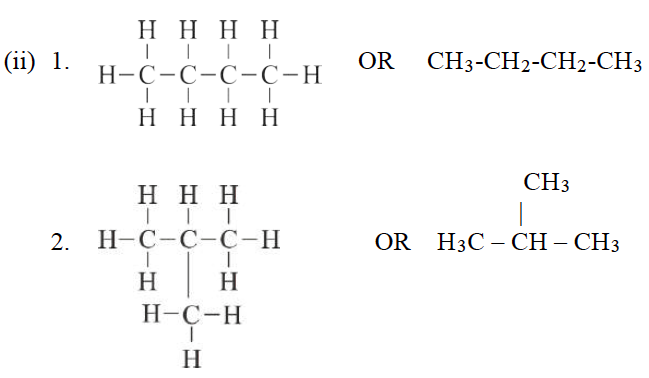(e) (i) White precipitate soluble in excess of sodium hydroxide solution.

(ii) Reddish brown vapour at the anode.

(iii) A white precipitate is formed which dissolves on heating or initially white precipitate is formed but on heating colourless solution is observed.

(iv) Colourless solution or solid CaCl2 turns into solution.

(v) A white precipitate is formed. (correct relevant observations accepted)

(f) (i) Oppositely charged ions are held by strong force of attraction or energy is required to separate the ions/ particles held by strong electrostatic force of attraction.

(ii) Inert gases have stable electronic configuration or have 8 electrons in the valence shell

(last shell) / do not lose or gain electrons to form ions/complete outermost or valence shell.

(iii) Nuclear charge increases / nucleus exerts greater force of attraction on the valence electrons of same shell or atomic size decreases / the force of attraction on the valence electrons are more i.e., increases.

(iv) Alkali metals have low ionization potential / have one electron in their valence shell /and hence they easily lose or donate electron.

(v) Dilute hydrochloric acid undergoes complete ionization/ dissociation whereas acetic acid undergoes incomplete or partial ionization or dilute HCl has higher concentration of ions than acetic acid./Dilute hydrochloric acid is a stronger acid than acetic acid/ Dilute hydrochloric acid is a strong electrolyte and acetic acid is a weak electrolyte/ Dilute hydrochloric -only ions, acetic acid- ions and molecules/ Dilute hydrochloric acid has higher degree of dissociation than acetic acid.

(g) (i) Nitrogen dioxide or NO2

(ii) Hydrogen sulphide or H2S

(iii) Nitric oxide / nitrogen monoxide or NO

(iv) Oxygen or O2

(v) Hydrogen or H2

(h)

(i) solid

(ii) hydrogen (gas)

(iii) upward

(iv) Haematite

(v) iron III chloride

### Question 2:

(a) (i) What do you understand by a lone pair of electrons?

(ii) Draw the electron dot diagram of Hydronium ion. (H=1; O=8)

(b) In Period 3 of the Periodic Table, element B is placed to the left of element A.

On the basis of this information, choose the correct word from the brackets to complete the following statements:

(i) The element B would have (lower / higher) metallic character than A.

(ii) The element A would probably have (lesser / higher) electron affinity than B.

(iii) The element A would have (greater / smaller) atomic size than B.

(c) Copy and complete the following table which refers to the conversion of ions to neutral particles.

 Conversion Ionic Equation Oxidation / Reduction Chloride ion to chlorine molecule (i) __________ (ii) __________ Lead (II) ion to lead (iii)__________ (iv) __________

(a) (i) The pair of electrons which is not yet shared with other atoms in a covalent molecule is known as lone pair of electrons.

(ii)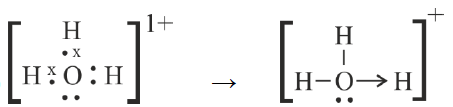(b) (i) higher

(ii) higher

(iii) smaller

(c) (i) 2Cl1- – 2e → Cl2 or Cl – e Cl (or Cl → Cl + e)

and Cl + Cl → Cl2 or 2Cl → Cl2

(ii) Oxidation

(iii) Pb2+ + 2e → Pb

(iv) Reduction

### Question 3:

(a) (i) Write the balanced chemical equation to prepare ammonia gas in the laboratory by using an alkali.

(ii) State why concentrated sulphuric acid is not used for drying ammonia gas.

(iii) Why is ammonia gas not collected over water?

(b) (i) Name the acid used for the preparation of hydrogen chloride gas in the laboratory. Why is this particular acid preferred to other acids?

(ii) Write the balanced chemical equation for the laboratory preparation of hydrogen chloride gas.

(c) For the preparation of hydrochloric acid in the laboratory:

(i) Why is direct absorption of hydrogen chloride gas in water not feasible?

(ii) What arrangement is done to dissolve hydrogen chloride gas in water?

(d) For the electro-refining of copper:

(i) What is the cathode made up of?

(ii) Write the reaction that takes place at the anode.

(a) (i)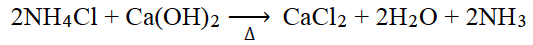(ii) Concentrated sulphuric acid reacts with ammonia / form ammonium sulphate or NH3 being basic combines with concentrated H2SO4 /correct chemical equation.

(iii) NH3 is highly soluble in water or dissolves in water.

(b) (i) Concentrate sulphuric acid.

It is nonvolatile / has high boiling point / displaces the volatile hydrogen chloride from

the salt sodium chloride.

(ii)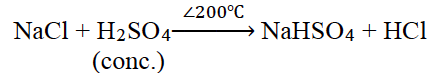(c) (i) Back suction occurs (or the description of back suction) / HCl gas dissolves in water at a faster rate than it is produced.

(ii) Inverted funnel arrangement/ the rim of the funnel just touches the surface of water taken in the trough or correct diagram.

(d) (i) Pure copper

(ii) Cu – 2e → Cu2+ / Cu → Cu2+ + 2e

### Question 4:

(a) The percentage composition of a gas is:

Nitrogen 82.35%, Hydrogen 17.64%.

Find the empirical formula of the gas. [N = 14, H = 1]

(b) Aluminum carbide reacts with water according to the following equation:

Al4C3 + 12H2O → 4Al (OH)3 + 3CH4

(i) What mass of aluminum hydroxide is formed from 12 g of aluminum carbide?

(ii) What volume of methane at s.t.p. is obtained from 12 g of aluminum carbide?

[Relative molecular weight of Al4C3 = 144; Al(OH)3 = 78]

(c) (i) If 150 cc of gas A contains X molecules, how many molecules of gas B will be present in 75 cc of B?

The gases A and B are under the same conditions of temperature and pressure.

(ii) Name the law on which the above problem is based.

(d) Name the main component of the following alloys:

(i) Brass

(ii) Duralumin

(a)

 % Composition Atomic Mass No. of atoms Simplest formula N 82.35 14 82.35/14=5.88 5.88/5.88=1 H 17.64 1 17.64/1=17.64 17.64/5.88=3

(b)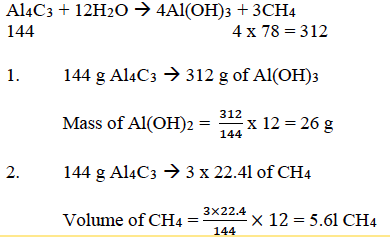(c) (i) No. of molecules of B = 75×𝑥/150 = 𝑥/2

(d) (i) Copper

(ii) Aluminum

### Question 5:

(a) Complete the following table which relates to the homologous series of hydrocarbons

 General formula IUPAC name of the homologous series Characteristic bond type IUPAC name of the first member of the series CnH2n–2 (A) __________ (B)__________ (C)__________ CnH2n +2 (D)__________ (E)__________ (F)__________

(b) (i) Name the most common ore of the metal aluminum from which the metal is extracted. Write the chemical formula of the ore.

(ii) Name the process by which impure ore of aluminum gets purified by using concentrated solution of an alkali.

(iii) Write the equation for the formation of aluminum at the cathode during the electrolysis of alumina.

(a) (A) Alkyne

(B) Triple covalent bond/ triple/≡ / C ≡ C/ CH ≡ CH

(C) Ethyne

(D) Alkane

(E) Single covalent bond/ single / − / C − C

(F) Methane

(b) (i) Bauxite Al2O3. 2H2O

(ii) Baeyer’s Process

(iii) Al3+ + 3e → Al

### Question 6:

(a) A compound X (having vinegar like smell) when treated with ethanol in the presence of the acid Z, gives a compound Y which has a fruity smell.

The reaction is: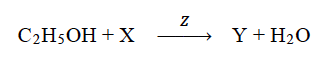(i) Identify Y and Z.

(ii) Write the structural formula of X.

(iii) Name the above reaction.

(b) Ethane burns in oxygen to form CO2 and H2O according to the equation:

2C2H6 + 7O2 → 4CO2 + 6H2O.

If 1250 cc of oxygen is burnt with 300 cc of ethane.

Calculate:

(i) the volume of CO2 formed.

(ii) the volume of unused O2.

(c) Three solutions P, Q and R have pH value of 3.5, 5.2 and 12.2 respectively. Which one of these is a:

(i) Weak acid?

(ii) Strong alkali?

(a) (i) Y: Ethyl acetate / Ethyl ethanoate / CH3COOC2H5

Z: Concentrated H2SO4

(ii)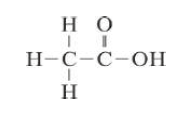(iii) Esterification

(b) (i) Volume of CO2 formed = 4/2 x 300 = 600 cc

(ii) Volume of O2 used = 7/2 x 300 = 1050 cc

Volume of unused oxygen = 1250 – 1050 = 200 cc

(c) (i) Solution Q

(ii) Solution R

### Question 7:

(a) Give a chemical test to distinguish between the following pairs of chemicals:

(i) Lead nitrate solution and Zinc nitrate solution

(ii) Sodium chloride solution and Sodium nitrate solution

(b) Write a balanced equation for the preparation of each of the following salts:

(i) Copper sulphate from Copper carbonate.

(ii) Zinc carbonate from Zinc sulphate.

(c) (i) What is the type of salt formed when the reactants are heated at a suitable

temperature for the preparation of Nitric acid?

(ii) State why for the preparation of Nitric acid, the complete apparatus is made up

of glass.

(d) Which property of sulphuric acid is shown by the reaction of concentrated sulphuric acid with:

(i) Ethanol?

(ii) Carbon?

(a) (i) By adding ammonium hydroxide in excess. Lead nitrate solution gives a white precipitate while Zinc Nitrate solution gives a colourless solution.

(ii) By adding silver nitrate / lead nitrate solution. Sodium chloride gives a white precipitate while sodium nitrate gives no precipitate / colourless solution / remains unchanged. (alternate correct chemical test)

(b) (i) CuCO3 + H2SO4 → CuSO4 + H2O +CO2

(ii) ZnSO4 + Na2CO3 → ZnCO3 + Na2SO4

Or

ZnSO4 + (NH4)2CO3 → (NH4)2SO4 + ZnCO3

(c) (i) Acid Salt/ bisulphate/ hydrogen sulphate/ NaHSO4/ KHSO4

(ii) The vapour of nitric acid being highly corrosive/ HNO3 vapours attack rubber, cork etc./ HNO3 vapours do not react with glass.

(d) (i) Dehydrating agent/dehydration

(ii) Oxidizing agent/oxidation

We hope students found this information on “ICSE Class 10 Chemistry Question Papers Solutions 2018” helpful for their exam preparation. They can also access solutions of other subjects of ICSE Class 10 Previous Years Question Papers by clicking here. Stay tuned to BYJU’S for the latest update on ICSE/CBSE/State Boards/Competitive exams. Also, download the BYJU’S App and fall in love with learning.